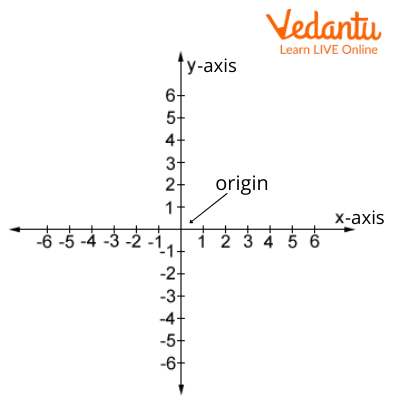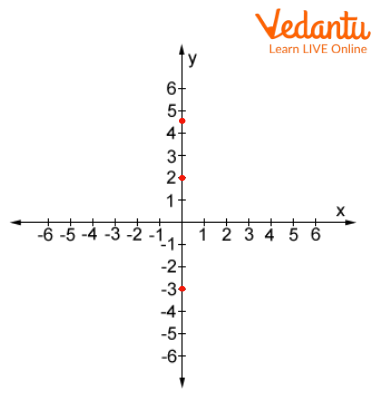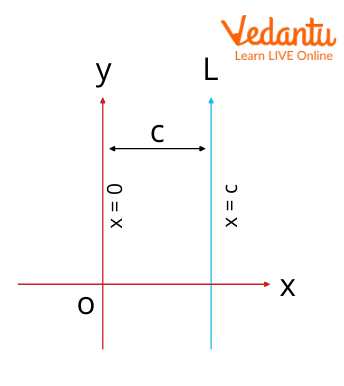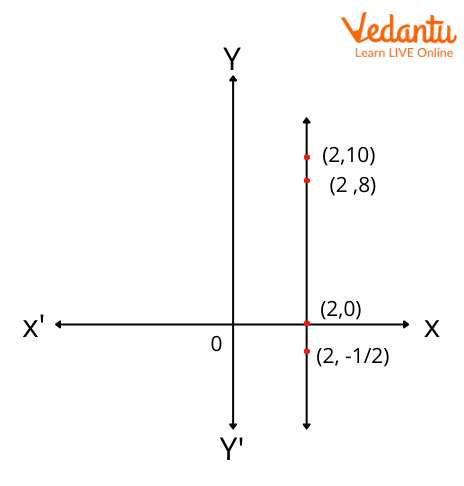Courses
Courses for Kids
Free study material
Free LIVE classes
More

# Equation of Y-axisLIVE
Join Vedantu’s FREE Mastercalss

To understand the equation of the y-axis. First, we have to understand graphs. What is a graph? A graph consists of a horizontal line and a vertical line, called the horizontal axis and the vertical axis, respectively. Why do we use a graph? A graph is used to represent data. A point can be determined horizontally or vertically, which can be easily understood using a graph. The horizontal axis is called the x-axis and the vertical axis is called the y-axis in a graph. Let us proceed further to know more about this topic.

## More about Graphs and X and Y-axis

To represent a point on a graph we take given points on the x-axis and y-axis respectively as (x,y). The (0,0) coordinate is called origin, where the x-axis and the y-axis meet. We take positive points at the x-axis and negative points at the x’-axis. Similarly, we take positive points at the y-axis and negative points at the y’-axis.A Graph

## What Is the Equation of the Y-axis?

Let us have an equation y = 2x + 3. To represent it, we will make a table.

 x y 0 3 1 5

Here we have values for both y and the x-axis. So, what is the equation of the y-axis?

When all the values of the equation lie on the y-axis, the values of x come 0 for all values of y, it is called the equation of the y-axis. So, the equation of y-axis is

x = 0

Let understand it with an example, x = 0*y

For y = 3, x will be 0

Y = -3, x will be 0

Similarly, for all value of y, x is 0.Graph of the Equation of Y-axis

## Line Parallel to Y-axis

A line is said to parallel to the y-axis when it is drawn along the y-axis, hence perpendicular to the x-axis, where the value of x remains constant for all values of y.

The common equation of the line parallel to the y-axis is

x= by + c, where b is 0

So, the equation of the line parallel to the y-axis is x = c. Here c is a constant.Graph of a Line Parallel to Y-axis

When b is 0, for all values of y, the value of x comes constant c. Let's understand it with an example.

x= (0)y + 2

 x y 3 2 3 0A Line parallel to the Y-axis

## Let’s Write the Equations of the Y-axis and X-axis

From the above discussion, we know the equation of the y-axis is x=0.

Similarly, the equation of the x-axis is y=0.

Here the point lies on the x-axis therefore for all values of x, y becomes 0. So, the equation of the y-axis is given by

x = 0

And the equation of the x-axis is given by

y = 0

## Questions for Practice

1. Draw the points (0,2), (0,4), and (0,-7)on a coordinate system. Do all the points lie on the same line? Can you name the line

1. Draw the points (2,0), (6,0), and (-3,0) on a coordinate system. Do all the points lie on the same line? Can you name the line?

1. Draw the points (3,2), (3,4.5), and (3,-3) on a coordinate system. Do all the points lie on the same line? Can you name the line?

(Answer: yes, a line parallel to the y-axis)

1. Draw the points (2,2), (0,2), and (6,2) on a coordinate system. Do all the points lie on the same line? Can you name the line?

(Answer: y a line parallel to the x-axis)

## Summary

We have learned the coordinates of the graph. The coordinate is written as (x,y).We have learned the equation of the y-axis. The equation is x = 0. We have learned the equation of the x-axis. The equation is y = 0. We have learned the equation of line parallel to the y-axis. The equation of the line parallel to the y-axis is x = c.

Last updated date: 22nd Sep 2023
Total views: 122.7k
Views today: 3.22k

## FAQs on Equation of Y-axis

1. What are the x-axis and y-axis?

The horizontal line in a graph is called the x-axis and the vertical line in a graph is called the y-axis. The point where these lines intersect is called the origin and its coordinate is (0,0). We represent points on a coordinate system as (x,y) where x represents the value of data on the x-axis and y represents the value of data on the y-axis. We can find the value of the coordinate x by drawing the perpendicular line to the x-axis. Similarly, we can find the value of coordinate y by drawing the line perpendicular to the y-axis.

2. How do you plot a graph with the x and y-axis?

At first, we draw and label the x and y-axis. Then we draw the coordinates of the function at various values of the x and y- coordinates. We draw lines perpendicular to the x-axis and the y-axis, where these lines intersect we draw a point and this is called (x,y) coordinates. Similarly, we draw all the coordinates then we connect all the coordinates and plot the graph of the function. To draw a graph we need a minimum two-point.